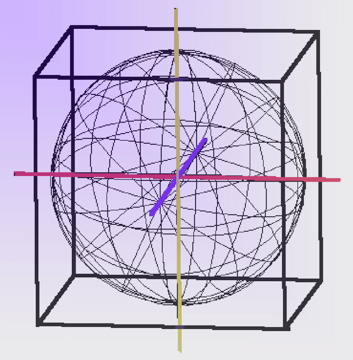# cube-sphereCubing the Sphere (as in Squaring the Circle) but in 3D

Sphere volume = 2*315*5153 = 147879835542

Cube volume = 65613 = 282429536481

Ratio: Sphere to Cube = Minor Seventh = 5:9::(2*315*5153):(65613)

3
3 X 2 = 6
3 X 3 = 9 (square)
3 X 4 = 12, etc.

31 = 3
32 = 9 (square)
33 = 27 (cube) 34 = 81 or

91 = 9 (32)
92 = 81 (32 X 32) (square made of 81 smaller squares)
93 = 729 (32 X 32 X 32) (cube made of 729 smaller cubes)

27 is the cube of 3. Think of a cube with 3 on each side. Such a cube can be broken up into 27 smaller cubes. 729 is the cube of 9. Think of a cube with 9 on each side. Such a cube can be broken up into 729 smaller cubes.

3 is the square root of 9. 3x3
3 is the cube root of 27. 3x3x3
9 is the square root of 81. 9x9
9 is the cube root of 729. 9x9x9

Music, arithmetic and geometry are three sides of the same triangle. Numbers 2 and 3 make up most musical notes until 5 is added in which makes everything wacko, but does fill in the missing notes. Let's do some more.....

Cube volume = diameter3

Sphere volume = 1/6 * PI * diameter3

A sphere enclosed in a cube means its diameter is the same as one side of the cube or we could say the sphere diameter = the cube root of the cube. Let's use 9 as our diameter, so:

Cube volume = 729

Sphere volume =
1/6 * PI * diameter3 =
1/6 * 3.14 * 93 =
1/6 * 3.14 * 243 =
3.14 * 243/6 =
763.02/6 = 127.17

If we did the same thing with 3 as diameter we have:

Cube volume = 27
Sphere volume = 14.13
Void = 27-14.13 or 12.87 parts of the cube

This all looks uninteresting until we remember that sound expands in spherical waves - NOT linear sine wave patterns. One series of base 2 is smaller cubes and spheres than a series of 3 base.

Musically this is something like:

27:14.13:: ninth
14.13:12.87:: seventh
27:12.87:: fourth?
Dale Pond
some references from Mechanical Engineer's Handbook, Lionel Marks, 5th edition, 1951.

Russell
"The entire birth and growth of matter matures at carbon. It matures as a flaming true sphere, any part of which will crystallize as a true cube when frozen into form. The cold cube of space and the hot sphere of matter are the consummate forms of Nature. Beyond that they cannot go. Also, they are Nature's limitations in pressures, temperatures and frequencies. Beyond the cube and the sphere they cannot go. When you understand this fundamental of Nature you will then understand WHY and HOW you and all things live, and WHY and HOW you die. Follow this explanation carefully then, and you will know. As to "WHERE you go when you die," that can wait, but that also you will know before you finish this book. We will leave this idea of cube-sphere limitation, and its reversal for a moment, but will return." [Atomic Suicide, page 34]

"The Creator follows conception with action and reaction. The inert gases divide and extend. Motion is then created for the purpose of giving body-forms to Idea. Divided and extended motion is expressed in pairs of light rings. Divided light-rings must necessarily divide the white Light of Mind into the tensions of the color spectrum. That means the red and blue of a bilateral universe which cannot exchange its sides, for each is interchangeably sex-conditioned. The sex strains and tensions are set up in the immovable rings of the inert gas of each octave, which is divided into pairs. Motion, tension, strain and heat begin with the extension of motion, in ring pairs, from its conception in the four recording rings of each octave. These four rings of the inert gases are within each other in the same plane. They are, therefore, two-dimensional. The moment they divide and extend into unit pairs, to simulate body forms, mass begins and the three dimensional, cube-sphere, bilateral universe appears. In other words neither God, nor man, create three-dimensional body-forms before conceiving the Mind-image of that form. Mind-images are thoughts. Body-images are actions, and actions are always in pairs. Thought-concepts are two-dimensional. Actions are polarized extensions, so actions are, necessarily, three-dimensional." [Atomic Suicide, page 228-229]